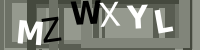# Oxide Formula

In ceramics, the chemistry of fired glazes is expressed as an oxide formula. There are direct links between the oxide chemistry and the fired physical properties.

## Details

Conceptually we consider fired ceramic glazes as being composed of 'oxides' (materials contribute these). Ten major oxides likely make up 99% of all base glazes (and materials we use). The oxide formula of a glaze "explains" many details about the way it fires (provided all the materials in the glaze dissolve in the melt). There is some redundancy with this term, sometimes people refer to "glaze formulas" when what they actually mean is "glaze recipes".

A formula expresses an oxide mix according to the relative numbers of molecule types (in contrast to a chemical analysis that compares them by weight). A formula is suited to analyzing and predicting properties of a fired glaze or glass because it gives us a picture of the molecular structure that is responsible for fired behavior. Since the kiln fires build these oxide molecules one-by-one into a structure, it follows that one will never fully 'understand' why a glaze fires the way it does without seeing its oxide formula.

Formulas are flexible. We can arbitrarily retotal one without affecting the relative numbers of oxide molecules. In fact, this retotaling of a formula is standard procedure to produce a Seger unity formula or a Mole% formula. With a formula, you need not worry whether there is 1 gram, 1 ton, or one billion molecules, only relative numbers matter. This is why it is allowable to express a formula showing molecule parts (e.g. 0.4 MgO; in reality this would not occur, but on paper a formula helps us compare relative numbers of oxide molecules in a ratio).

An example of a raw formula:

 FluxesRO IntermediatesR2O3 Glass FormersRO2 K2OCaOMgOZnO 0.51.30.20.1 Al2O3 0.9 SiO2 9.0

Notice in the above that oxides are grouped into three columns: the bases, acids, and amphoterics (or simply as the RO, R2O3, and RO2 oxides; where "R" is the element combining with oxygen). Actually, the ratio of R to O is significant. The right column has the greatest oxygen component, the left has the least. Simplistically, we can view these three groups as the silica:alumina:fluxes system. This latter convention is not really correct because there are more glass builders than SiO2 , other intermediates besides Al2O3, and the RO's do more than just flux. Ancient potters referred to these three as the blood, flesh, and bones of a glaze (not a bad way to think of it).

Any formula has a formula weight, that is, the total calculated weight for that mix of molecules. Atomic weights are published in any ceramic text so it is easy to calculate the weight of each oxide. Calculating the weight of the whole is just a matter of simple addition and then increasing that weight to account to LOI.

Insight-live normally determines the unity of formulas it calculates automatically. If the RO oxides total is greater than R2O3/2 it is deemed flux unity. Otherwise it is R2O3 unity.

## Related Information

### Insight-Live comparing a glossy and matte cone 6 base glaze recipe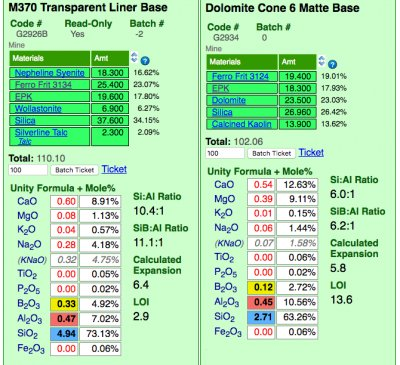Insight-live is calculating the unity formula and mole% formula for the two glazes. Notice how different the formula and mole% are for each (the former compares relative numbers of molecules, the latter their weights). The predominant oxides are very different. The calculation is accurate because all materials in the recipe are linked (clickable to view to the right). Notice the Si:Al Ratio: The matte is much lower. Notice the calculated thermal expansion: The matte is much lower because of its high levels of MgO (low expansion) and low levels of KNaO (high expansion). Notice the LOI: The matte is much higher because it contains significant dolomite.

### A limit or target glaze formula. What does this mean?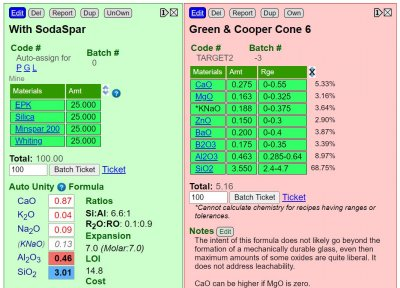Recipes show us the materials in a the glaze powder (or slurry). Formulas enumerate the oxide molecules and their comparative quantities in the fired glass. Oxides construct the fired glass. The kiln de-constructs ceramic materials to get their oxides, discards the carbon, sulfur, etc. and builds the glass from the rest. There is a direct relationship between fired glaze properties (e.g. melting range, gloss, thermal expansion, hardness, durability, color response, etc) and its oxide formula. There are 8-10 oxides to know about (vs. hundreds of materials). From the formula-viewpoint materials are thus "sources-of-oxides". While there are other factors besides pure chemistry that determine how a glaze fires, none is as important. Insight-live can calculate and show the formula of a recipe, this enables comparing it side-by-side and with a target formula (or another recipe known to work as needed). Target formulas are opened using the advanced recipe search, choosing the limits batch and clicking/tapping the search button (search 'target recipe' in Insight-live help for more info).

### A Limitation of the Seger Unity Formula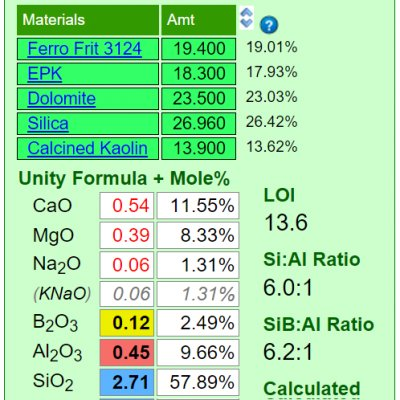Mineral sources of oxides impose their own melting patterns and when one is substituted for another to supply an oxide a different system with its own relative chemistry is entered. An extreme example of this would be to source Al2O3 to a glaze using calcined alumina instead of kaolin. Although the formula may be exactly the same, the fired result would be completely different because very little of the alumina would dissolve into the glaze melt. At the opposite extreme, a different frit could be used to supply a set of oxides (while maintaining the overall chemistry of the glaze) and the fired result would be much more chemically predictable. Why? Because the readily and release their oxides the the melt.

### Ceramic Oxide Periodic TableAll common traditional ceramic base glazes are made from only a dozen elements (plus oxygen). Materials decompose when glazes melt, sourcing these elements in oxide form. The kiln builds the glaze from these, it does not care what material sources what oxide (assuming, of course, that all materials do melt or dissolve completely into the melt to release those oxides). Each of these oxides contributes specific properties to the glass. So, you can look at a formula and make a good prediction of the properties of the fired glaze. And know what specific oxide to increase or decrease to move a property in a given direction (e.g. melting behavior, hardness, durability, thermal expansion, color, gloss, crystallization). And know about how they interact (affecting each other). This is powerful. And it is simpler than looking at glazes as recipes of hundreds of different materials (each sources multiple oxides so adjusting it affects multiple properties).

### Desktop INSIGHT showing formula and analysis side-by-side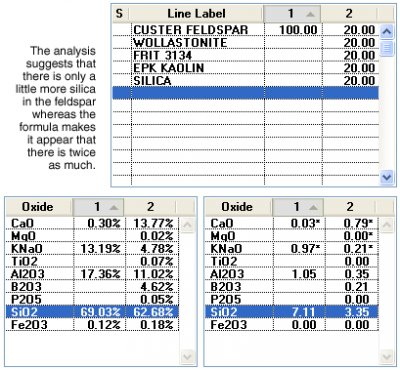Desktop Insight was the first to enable users to compare two recipes and their formulas side-by-side and interactively update when recipe changes were made. It also enabled users to show formulas and analyses side-by-side.

### Example of a whole rock chemical analysis lab report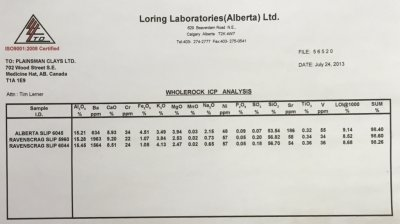Powdered samples were sent to the lab. The numbers shown on this report are in percentage-by-weight. That means, for example, that 15.21% of the weight of the dry powder of Alberta Slip is Al2O3. Insight-live knows material chemistries in this way (whereas desktop Insight needs them as formulas). Some non-oxide elements are quantified as parts-per-million (these amounts are not normally high enough to take into account for traditional ceramic purposes). The LOI column shows how much mechanically and chemically bound water are gassed off during firing of the sample. The total is not exactly 100 because of inherent error in the method and compounds not included in the report.

Glossary Formula Weight Chemical Analysis In ceramics, raw material chemistry is expressed a chemical analyses. This is in contrast to fired glaze chemistries which are expressed as oxide formulas. Frit Frits are used in ceramic glazes for a wide range of reasons. They are man-made materials of controlled chemistry with many advantages or raw materials. Unity Formula The chemistry of ceramic glazes are normally expressed as formulas. A unity formula has been retotaled to make the numbers of flux oxides total one. Ceramic Oxide In glaze chemistry, the oxide is the basic unit of formulas and analyses. Knowledge of what materials supply an oxide and of how it affects the fired glass or glaze is a key to control. KNaO - Potassium/Sodium Oxides Substituting Materials by Weight: Why it does not work! Wollastonite is 50:50 CaO:SiO2. So why not just substitute 40 wollastonite for 20 calcium carbonate and 20 silica? The answer will help you see reason why we make such a big deal of glaze chemistry. Desktop Insight 5A - Glaze Formula to Batch Calculations Learn to use a non-unity calculation to convert a formula into a batch recipe using theoretical and real-world materials. Retotal, round-off and make a side-by-side report. Desktop Insight 1A - Compare Theoretical and Real-World Feldspars While comparing a real-world and theoretical feldspar learn to enter, edit, save, normalize recipes and the materials dialog. Glaze chemistry concepts. How desktop INSIGHT Deals With Unity, LOI and Formula Weight INSIGHT enables you to enter material analyses as recipes. This is a first step to inserting them into the materials database. Imposing an LOI and understanding how to set unity, and its connection with formula weight are important concepts. Glaze Chemistry Basics - Formula, Analysis, Mole%, Unity, LOI Part of changing your viewpoint of glazes, from a collection of materials to a collection of oxides, is learning what a formula and analysis are, how conversion between the two is done and how unity and LOI impact this.

Name

Subject

Message